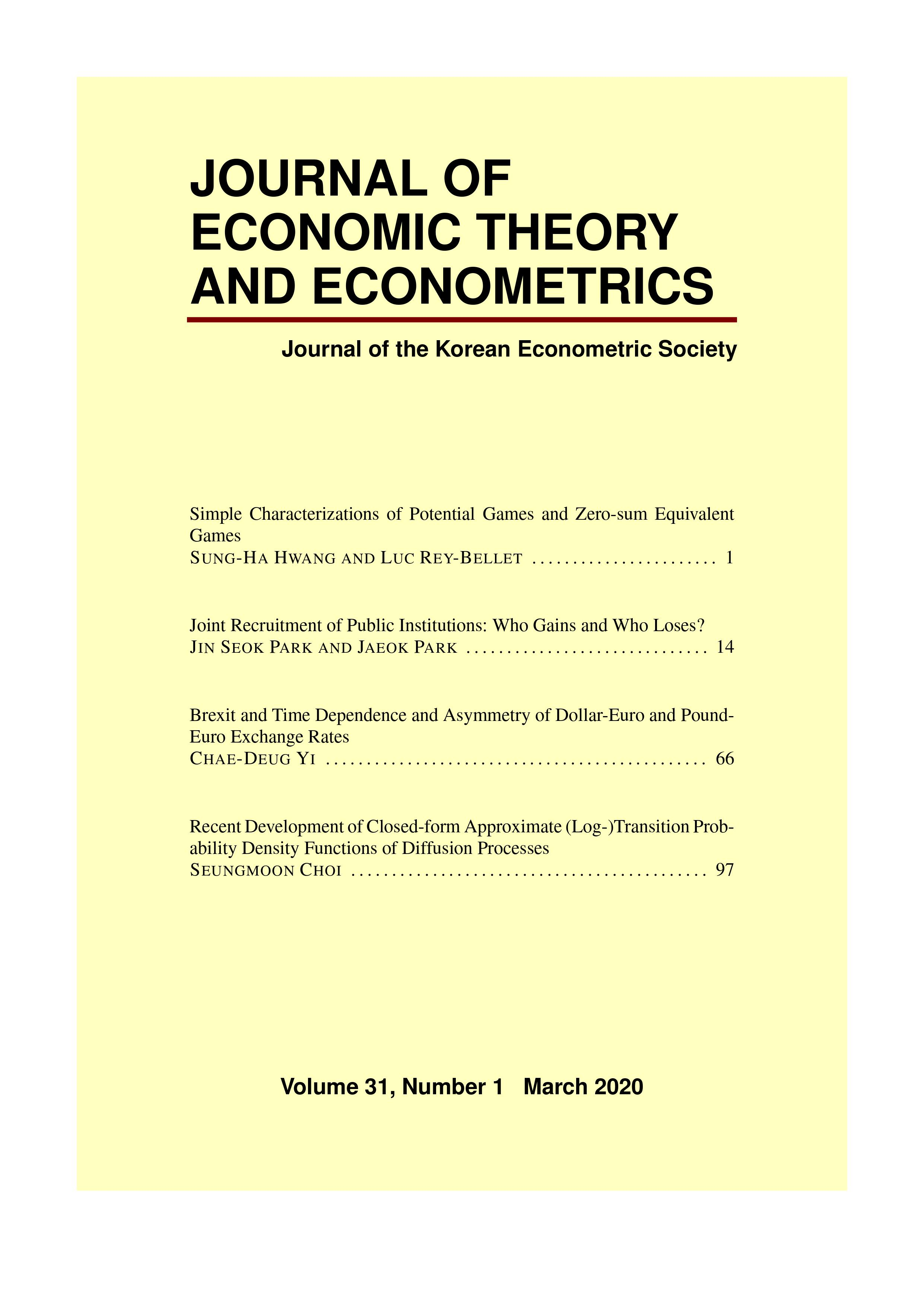상세검색
최근 검색어 전체 삭제
다국어입력
즐겨찾기0KCI등재 학술저널

# Simple Characterizations of Potential Games and Zero-sum Equivalent Games

• 등재여부 : KCI등재
• 2020.03
• 1 - 13 (13 pages)
• 5

We provide several tests to determine whether a game is a potential game or whether it is a zero-sum equivalent game&#8212;a game which is strategically equivalent to a zero-sum game in the same way that a potential game is strategically equivalent to a common interest game. We present a unified framework applicable for both potential and zero-sum equivalent games by deriving a simple but useful characterization of these games. This allows us to re-derive known criteria for potential games, as well as obtain several new criteria. In particular, we prove (1) new integral tests for potential games and for zero-sum equivalent games, (2) a new derivative test for zero-sum equivalent games, and (3) a new representation characterization for zero-sum equivalent games.

1. INTRODUCTION

2. MAIN RESULTS

3. EXAMPLES

4. DISCUSSION